# ORF523: Acceleration by randomization for a sum of smooth and strongly convex functions

In this lecture we are interested in the following problem: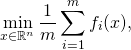where each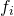is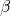-smooth and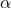strongly-convex. We denote bythe condition number of these functions (which is also the condition number of the average).

Using a gradient descent directly on the sum with step-sizeone obtains a rate of convergence of orderfor some numerical constant(see this lecture). In particular one can reach an-optimal point in timewith this method, where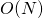is the time to compute the gradient of a single function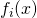. Using Nesterov’s Accelerated Gradient Descent (see this lecture) one can reduce the time complexity to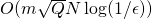.

Using the SGD method described in the previous lecture (with the step-size/averaging described here) one can reach an-optimal point in time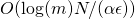which is much worse than the basic gradient descent if one is looking for a fairly accurate solution. We are interested in designing a randomized algorithm in the spirit of SGD that would leverage the smoothness of the function to attain a linear rate of convergence (i.e., the dependency inwould be of the form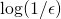).

We first describe the SAG method proposed in this paper by Le Roux, Schmidt and Bach (2012). The method is simple and elegant: let,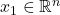and forletbe drawn uniformly at random inand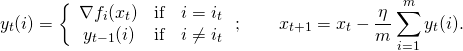They show that in the regime where, SAG (with step-sizeand with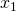obtained as the average of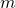iterates of SGD) has a rate of convergence of order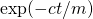(see Proposition 2 in their paper for a more precise statement). Since each step has a time-complexity ofthis results in an overall time-complexity of, which is much better than the complexity of the plain gradient descent (or even of Nesterov’s Accelerated Gradient Descent). Unfortunately the proof of this result is quite difficult. We will now describe another strategy with similar performance but with a much simpler analysis.

Short detour through Fenchel’s duality

Before describing the next strategy we first recall a few basic facts about Fenchel duality. First the Fenchel conjugate of a function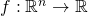is defined by: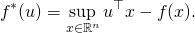Note that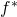is always a convex function (possibly taking value). Ifis convex, then for any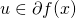one clearly has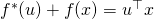. Finally one can show that ifis-smooth thenis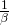-strongly convex (one can use the second Lemma from this lecture).

Stochastic Dual Coordinate Ascent (SDCA)

We describe now the SDCA method proposed in this paper by Shalev-Shwartz and Zhang. We need to make a few more assumptions on the functions in the sum, and precisely we will now assume that we want to solve the following problem (note that all norms here are Euclidean):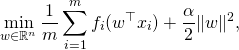where

•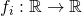is convex,-smooth.
•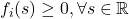and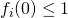.
•.

Denote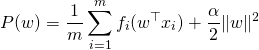the primal objective. For the dual objective we introduce a dual variable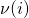for each data point(as well as a temporary primal variable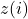):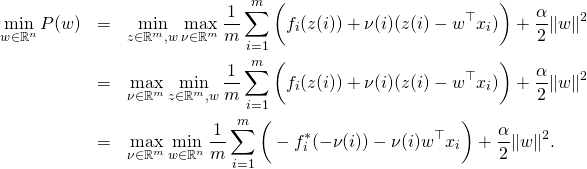Next observe that by the KKT conditions one has at the optimum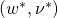:and thus denoting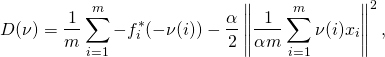we just proved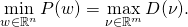We can now describe SDCA: letand for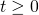letbe drawn uniformly at random inand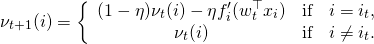Letbe the corresponding primal variable. The following result justifies the procedure.

Theorem (Shalev-Shwartz and Zhang 2013) SDCA with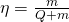satisfies: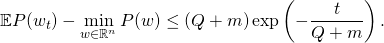The above convergence rate yields an overall time-complexity of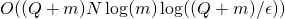for SDCA (whereis the complexity of computing a gradient of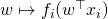), which can be much better than thecomplexity of the plain gradient descent.

Proof: The key property that we will prove is that the primal/dual gap is controlled by the improvement in the dual objective, precisely:

(1)Let us see how the above inequality yields the result. First we clearly have, and thus the above inequality immediately yields: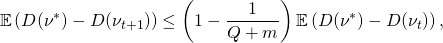which by induction gives (usingand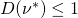):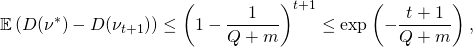and thus using once more (1):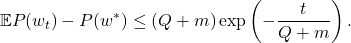Thus it only remains to prove (1). First observe that the primal/dual gap can be written as:Let us now evaluate the improvement in the dual objective assuming that coordinateis updated in thestep: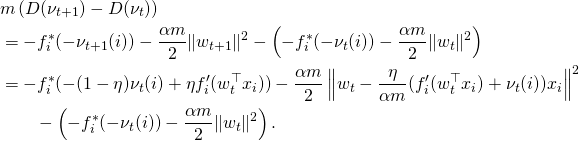Now using thatis-strongly convex one haswhich yieldswhere in the last line we used thatand. Observe now thatwhich finally givesand thus taking expectation one obtains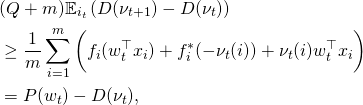which concludes the proof of (1) and the proof of the theorem.This entry was posted in Optimization. Bookmark the permalink.

## 2 Responses to "ORF523: Acceleration by randomization for a sum of smooth and strongly convex functions"

••